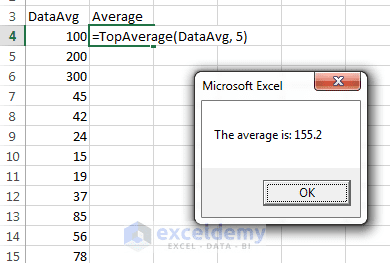Disclosure: This post may contain affiliate links, meaning when you click the links and make a purchase, we receive a commission.

# An Excel VBA function with a range argument

Well, the last type of argument is a range. It is demonstrated here.

## An Excel VBA function with a range argument

Let’s make some assumption. Say, you have some values. The number of values may be 100, 1000, or any. Your boss has told you to give him the average of largest 5 values of this data. What can you do? First, you’ll think that whether there is any predefined function in Excel to solve it efficiently. Yes, it has a LARGE function. The LARGE function does not give the result directly. You have to use it trickily. You can use the LARGE function in the following way (assuming that you have 20 values and the data range is named “DataAvg”):

```=(LARGE(DataAvg, 1)+LARGE(DataAvg, 2)+LARGE(DataAvg, 3)+
LARGE(DataAvg, 4)+LARGE(DataAvg, 5))/5```

The above formula will return the average of the largest 5 values of your data. The formula works fine, but you will face a problem when next time your boss will ask you to average of 100 values from a data range of say 2000 values. This time you have to rewrite this formula in a new way and it is really toilsome. Here comes the custom VBA function, TopAverage (DatAvg, Num).Average is showed in a message box.

I have written the TopAverage function in the following way:

```Function TopAverage(DataAvg, Num)
'Returns the average of the highest Num values in Data
Sum = 0
For i = 1 To Num
Sum = Sum + WorksheetFunction.Large(DataAvg, i)
'Large(Data, i) returns the ith largest value from the Data.
Next i
TopAverage = Sum / Num
MsgBox "The average is: " & TopAverage
End Function
```

This situation is an example of how a custom function can make things easier for you. This function takes two arguments: DataAvg and Num. DataAvg represents the range of data that you’re working with. Num is the number of largest values you want to average from the data range.

The code initializes the Sum variable to 0. Then it uses For- Next loop to calculate the sum of the Num number largest values. Notice that I have used the built-in LARGE function of Excel in the code. You can use any worksheet function in the custom VBA function using WorksheetFunction and a period before the function name (WorksheetFunction.LARGE).

Finally, the average is found just dividing the Sum by the Num (Sum/Num). I showed the result in a message box with this text: “The average is: “.